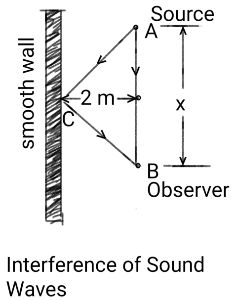# The lecturer with a monotone voice of 440 Hz and you are both 2m away from the long, smooth and...

## Question:

The lecturer with a monotone voice of 440 Hz and you are both 2m away from the long, smooth and tall wall. You will hear the sound that comes directly to you from the lecturer along with sound that comes back from the wall on its way to reaching you. You need the path here that needs to have the same angle onto the wall as it has after reflection. How far from the lecturer will you pick your seat so you cannot hear the voice at all and find your well-deserved rest?

## Interference of Two Waves:

Interference is a phenomenon of superposition of two identical waves which reach a point with a path difference between them, resulting in an increase in intensity if the waves reach the point in phase and zero intensity if the waves reach the point with a phase difference {eq}180^\circ {/eq}.

Let us consider the following two waves:

{eq}\displaystyle{y_1 = y_0 \sin\left[\dfrac{2\pi}{\lambda} (Vt - x)\right]\\ y_2 = y_0 \sin\left[\dfrac{2\pi}{\lambda}(Vt - (x + \delta))\right]} {/eq}.

{eq}\delta {/eq} is the path difference between the identical waves.

As the waves superpose, the equation of the resultant wave can be given by:

{eq}\displaystyle{Y = 2y_0 \cos\left(\dfrac{\pi\delta}{\lambda}\right) \sin\left[\dfrac{2\pi}{\lambda}\left(Vt - \left(x - \dfrac{\delta}{2}\right)\right)\right]} {/eq}.

The amplitude of the resultant wave will be given by:

{eq}\displaystyle{Y_0 = 2y_0 \cos\left(\dfrac{\pi\delta}{\lambda}\right)} {/eq}.

If the path difference {eq}(\delta) {/eq} is equal to zero or the even multiple of {eq}\dfrac{\lambda}{2} {/eq}, there will be constructive interference and if the same is the odd multiple of {eq}\dfrac{\lambda}{2} {/eq}, there will be destructive interference.

In the case of constructive interference of two sound waves, there will be an intensified sound much louder than each of the original sounds and in the case of destructive interference of two sound waves, there will be a silence observed.

Corresponding DiagramLet the lecturer be standing at A and me, the observer, be standing at B. Let AB {eq}= x {/eq}. C is the point of reflection of the sound wave. The sound waves reach from the source, the lecturer, to the observer in two ways - directly in AB path and after reflection at O through AC and CB paths.

The distance of the source and the observer from the smooth wall is {eq}2 \ \rm m {/eq}.

Given

• The frequency of the monotone: {eq}\nu = 440 \ \rm Hz {/eq}.

Known

• The speed of sound: {eq}V = 332 \ \rm m/s {/eq}.

Therefore, the wavelength of the sound wave is given by:

{eq}\displaystyle{\begin{align*} \lambda &= \dfrac{V}{\nu}\\ \lambda &= \dfrac{332 \ \rm m/s}{440 \ \rm Hz}\\ \lambda &\approx 0.754 \ \rm m\\ \end{align*}} {/eq}

The minimum path difference between the two sound waves to meet the condition of destructive interference is given by:

{eq}\displaystyle{\begin{align*} \delta_{min} &= \dfrac{\lambda}{2}\\ \delta_{min} &= \dfrac{0.754 \ \rm m}{2}\\ \delta_{min} &= 0.377 \ \rm m\\ \end{align*}} {/eq}

From the diagram, we get:

{eq}\displaystyle{(AC + CB) - AB = 2\times\sqrt{\dfrac{x^2}{4} + 4 \ \rm m^2} - x} {/eq}.

Then, we write:

{eq}\displaystyle{\sqrt{\dfrac{x^2}{4} + 4 \ \rm m^2} - x = \delta_{min} = 0.377 \ \rm m \ \ \ \ (1)} {/eq}.

Solving (1), we have the minimum distance between the lecturer and the observer (so that observer may not here the lecturer's lecture) given by:

{eq}\displaystyle{x = 1.820 \ \rm m} {/eq} (neglecting the negative root of the quadratic equation).